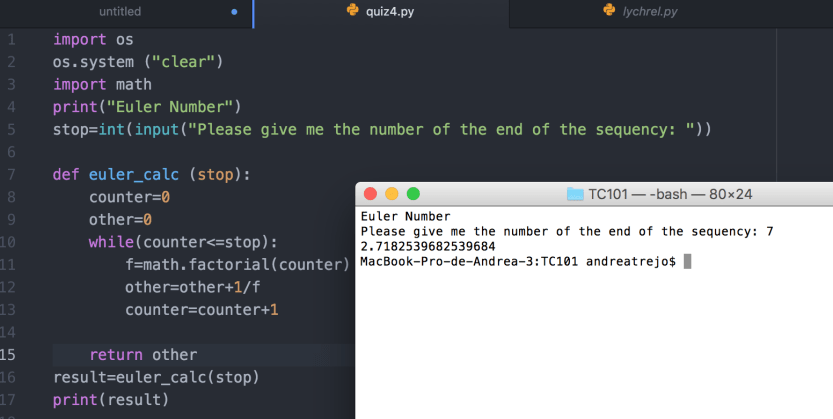This was my face when I realized I was missing Quiz #4 too:The number e is an important mathematical constant that is the base of the natural logarithm. It is approximately equal to 2.71828, and is the limit of (1 + 1/n)n as n approaches infinity, an expression that arises in the study of compound interest. It can also be calculated as the sum of the infinite series.Create a function called euler_calc with a single parameter precision. The value of                  precision is used to determine when to stop calculating. Your calculation will stop                  when the two consecutive values estimating e differ by less than precision (remember          to use absolute value when calculating the difference between two values here).

So when I finished reading this, my face was just like WTH is this, so I did my research and I found some interesting stuff, because I didn’t understand a thing about this quiz haha.

In order to understand a little bit more of this number  you can check this link out, it explains perfectly what this number is about, so you can do your program better,and one way to know if the program is working correctly is knowing that the maximum value of the e number is:2.71828…so basically the user have to give you the parameter and the program will stop when this is between 2 numbers I think :S.

So here’s the screenshot of the program and the code in GitHub